Back to blog

# Christmas worksheets: maths

Maths

Dec 6, 2022, 4:35 PM## Contents

Looking for fun, festive educational activities for your child this winter? We hope ‘yule’ enjoy these free, printable Christmas-themed maths worksheets!

Each worksheet includes ten jolly multiplication and division questions. Your child can practise knowledge and skills from the Key Stage 2 maths national curriculum. The answers are included at the end of each worksheet.

## Age 7–8 (Year 3)

Practise mental multiplication and division:## Age 8–9 (Year 4)

Practise mental multiplication and division: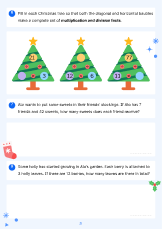## Age 9–10 (Year 5)

Practise mental multiplication and division: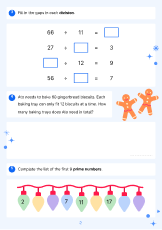## Age 10–11 (Year 6)

Practise long division, long multiplication and mental arithmetic: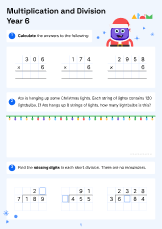More free maths resources

## Useful maths videos

These maths videos will help your child with the skills and knowledge needed for the activities above.

### Introduction to multiplication tutorial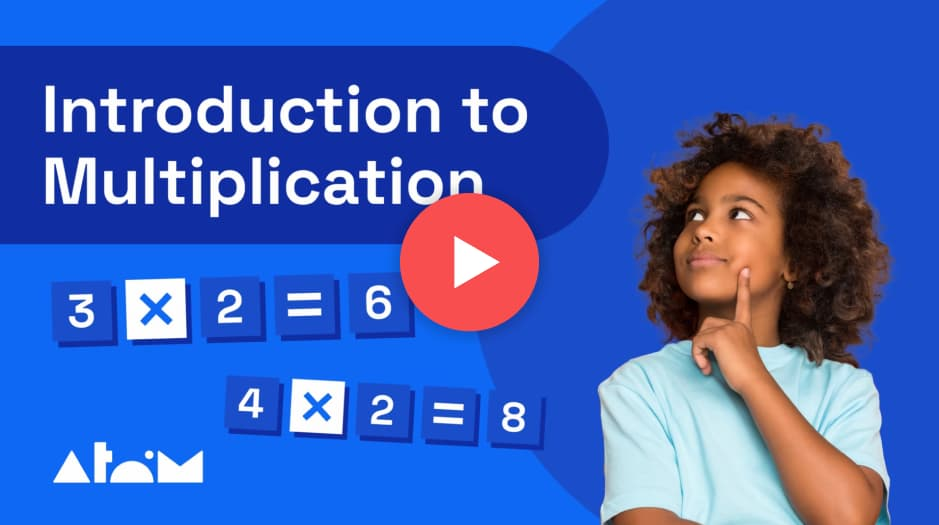In this tutorial, learn what multiplication is with step-by-step examples.

Multiplication is the calculation we use to express repeated additions of equal groups. Using repeated addition will always help you find the answer to a multiplication – but it is much quicker to learn your times tables by heart!

### Short division tutorial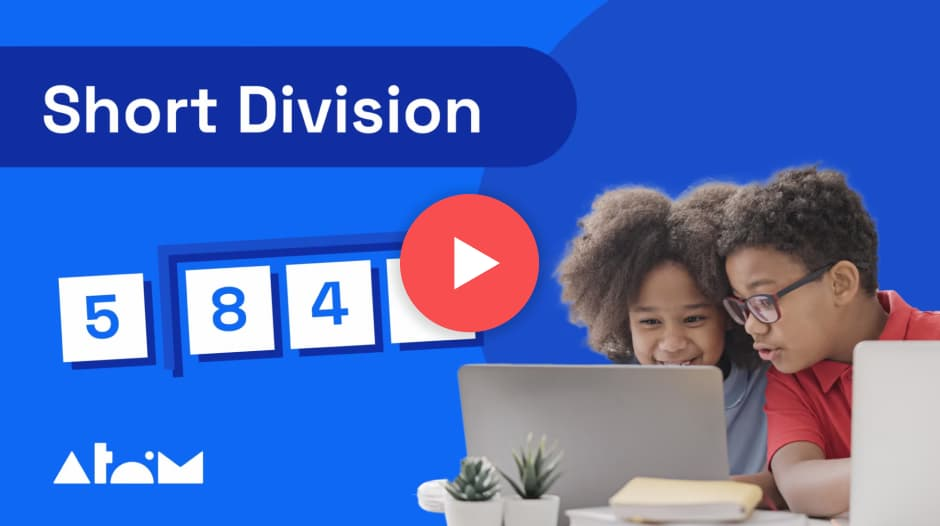In this tutorial, learn how to divide 3-digit numbers using short division. You'll see a step-by-step worked example of a short division problem.

Division is the calculation used to share an amount into equal groups. Short division is used to divide large numbers by 1-digit numbers. You may know this as the 'bus stop method' as the lines look a little like a bus stop.

### Long division tutorial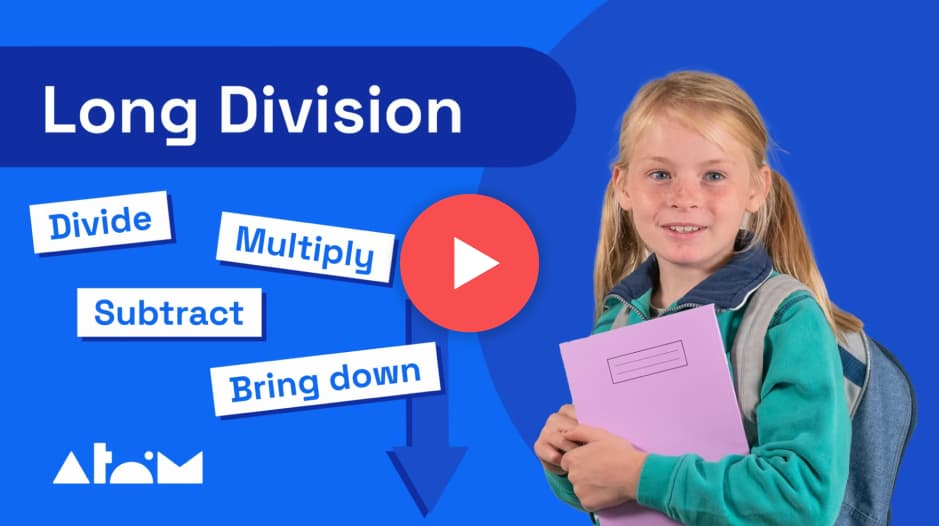In this tutorial, learn how to divide larger numbers using long division. You'll see a step-by-step worked example of a long division problem.

Use the mnemonic Dangerous Monkeys Steal Bananas to remember the steps for long division:

1. Divide

2. Multiply

3. Bring down

4. Subtract

## Make the most of online learning this holiday

Looking for more fun, interactive ways for your child to learn maths?

Atom Nucleus is the all-in-one online learning platform to help children build knowledge and confidence in key subjects and prepare for exams.• Over 70,000 interactive questions in English, maths, and science

• Automatic marking with data insights, allowing you to stay on top of your child's progress

• Hundreds of engaging helpsheets and video lessons

With Nucleus your child can work independently, exploring exciting worlds as they master the Key Stage 2 national curriculum.

## More holiday activities for your childAtom

Learning